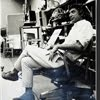## Eugene Ray SDSU 1970'sEugene Ray 1970's. photo credit: Tom Davis

## Sunday, November 11, 2012

### POWER OF THE TOWER gives us deep GENETIC MEMORY feeling !^^^^^^^^^^^^^^^^^^^^^ ROUND TOWERING STRUCTURE> is nutrient for my inherited favor ^^^^^^^^^^^^^^^^^^^^^ RADIANT ARCHITECTURE reveals seligman residence as early star> ^^^^^^^^^^^^^^^^^^^^^ PHOTOS PROVIDE EVIDENCE >> VERTICAL STRONG tower image ^^^^^^^^^^^^^^^^^^^^^ (tower memory in many designs from my oeuvre louisiana/calif ) Inbox+ROUND TOWER POWER+
>historical progenitor<
^^^^^^^^^^
>reviewing my architectural oeuvre<
>reveals inherited memory as a <
+VITAL FORCE+
>in the residential architecture<
^^^^^^^^^^^^^^^^^

+ROUND TOWERS+
>have played an enormous role in<
>the castles of our ancestors and<
^^^^^^^^^^^^^^^^^^
+PROVIDE MUCH+
+BIOTRONIC ENERGY+
^^^^^^^^^^
^^^^^^^^^^^^^^
+EUGENE RAY / DEGRUY+
+ROUND TOWERS+
^^^^^^^^^
>to review previous thoughts<
>on this vital, seminal topic<
^^^^^^^^^^^^^

+TONIGHT'S PHOTOS+
^^^^^^^^^^^

#1)       +SELIGMAN RESIDENCE+
>the largest of my louisiana houses<
>expresses the vertical austerity<
>of castelated towers and<
^^^^^^^^^^^^^^^
+COSMIC POWER+
^^^^^^^^^

+SELIGMAN DRAWINGS+
>the original drawings now at the<
+HISTORIC NEW ORLEANS+
+C O L L E C T I O N+
>studied carefully reveal strength<
>in the round austere elevation<
>evoking ancient memories<
^^^^^^^^^^^^^^

#2)           +DOUBLE TOWERS+
>seen in these two residence designs<
>remind us of the silver ship design<
>which has one 25 ft diameter<
>tower split in half and<
^^^^^^^^^^^
>with a giant 10 ft by 40 ft truss<
>providing two upper floors<
+STRONG-LIGHTED+
>forming the roof vault<
^^^^^^^^^^^^

#3)          +GRUYERE CASTLE+
>of my de gruy(ere) ancestors has an<
+ANCIENT TOWER+
>as its original residence keep that<
>is forever engraved in my<
+METAPHYSICAL+
>inherited dna psychic memory<
>indelible/strong/compelling<
^^^^^^^^^^^^^^^

#4)        +EGYPTIAN TOWERS+
>egyptian and sumerian towers take<
>us back to extraterrestrial vision<
+SO OLD ITS IN THE FUTURE+
^^^^^^^^^^^^^^^
>nourishing and bright<
^^^^^^^^^^^^
#5)         +ROUND TOWERS+
>of the pierucci solar heated residence<
>express energy of the sun as well as<
+BIOTRONIC ENERGY+
>silent organic and eternal<
^^^^^^^^^^^^^^

+FLASH OF LIGHT+
>in the<
+EVENING SKY+
^^^^^^^

gene ray, lajolla, california

>eugene ray, mfa, architect<
>professor emeritus, sdsu<
^^^^^^^^^^^^^